## Sherlin and Danju are playing Ludo at home during Covid-19. While rolling the dice, Sherlin’s sister Raji observed and noted the possible outcomes of the throw every time belongs to set {1, 2, 3, 4, 5, 6}. Let A be the set of players while B be the set of all possible outcomes.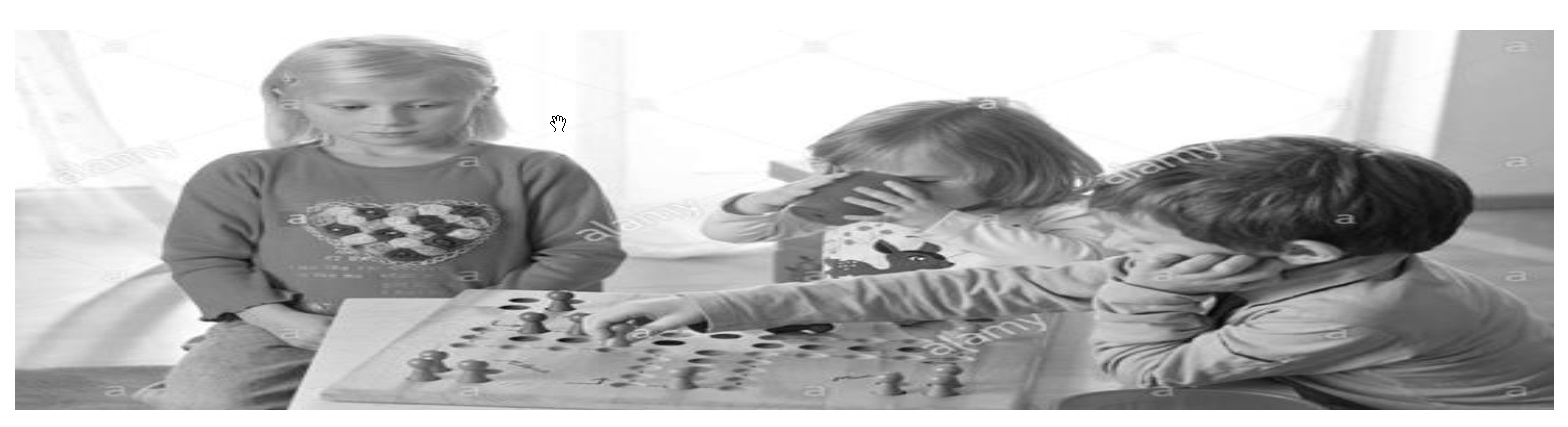(adsbygoogle = window.adsbygoogle || []).push({}); A = {S, D}, B = {1, 2, 3, 4, 5, 6}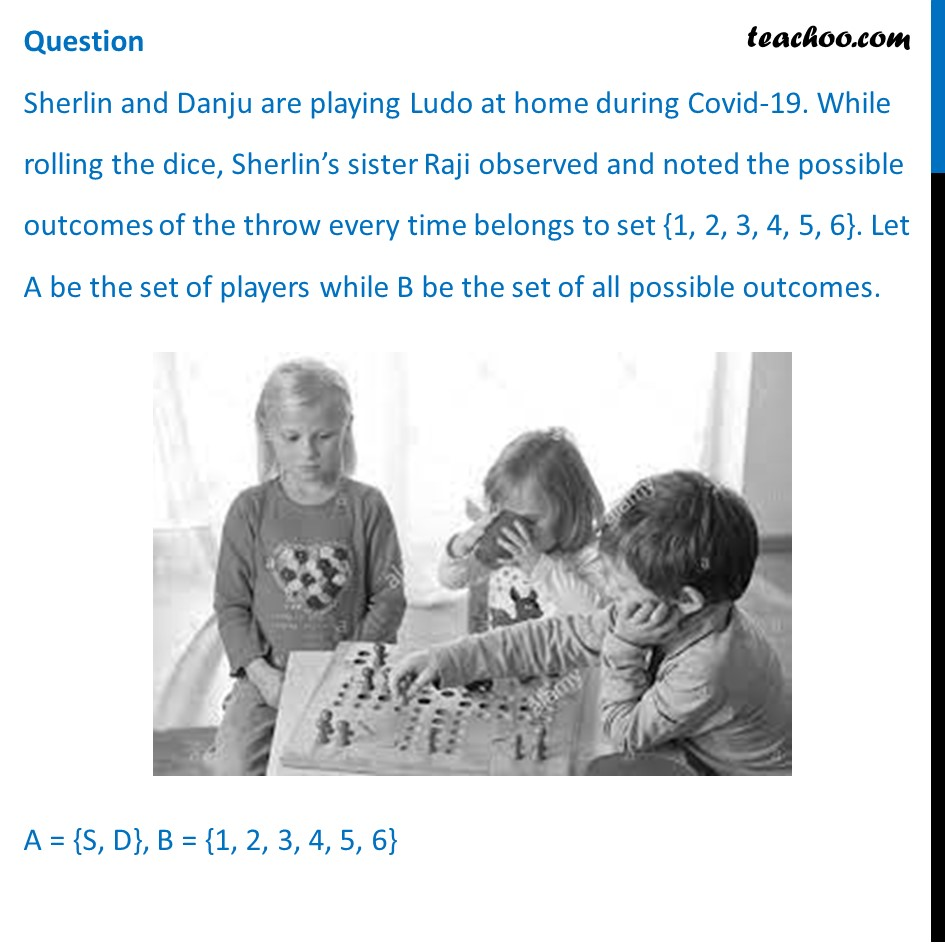## Let 𝑅 ∶ 𝐵 → 𝐵 be defined by R = {(𝑥, 𝑦): 𝑦 𝑖𝑠 𝑑𝑖𝑣𝑖𝑠𝑖𝑏𝑙𝑒 𝑏𝑦 𝑥 } is (a) Reflexive and transitive but not symmetric (b) Reflexive and symmetric and not transitive (c) Not reflexive but symmetric and transitive (d) Equivalence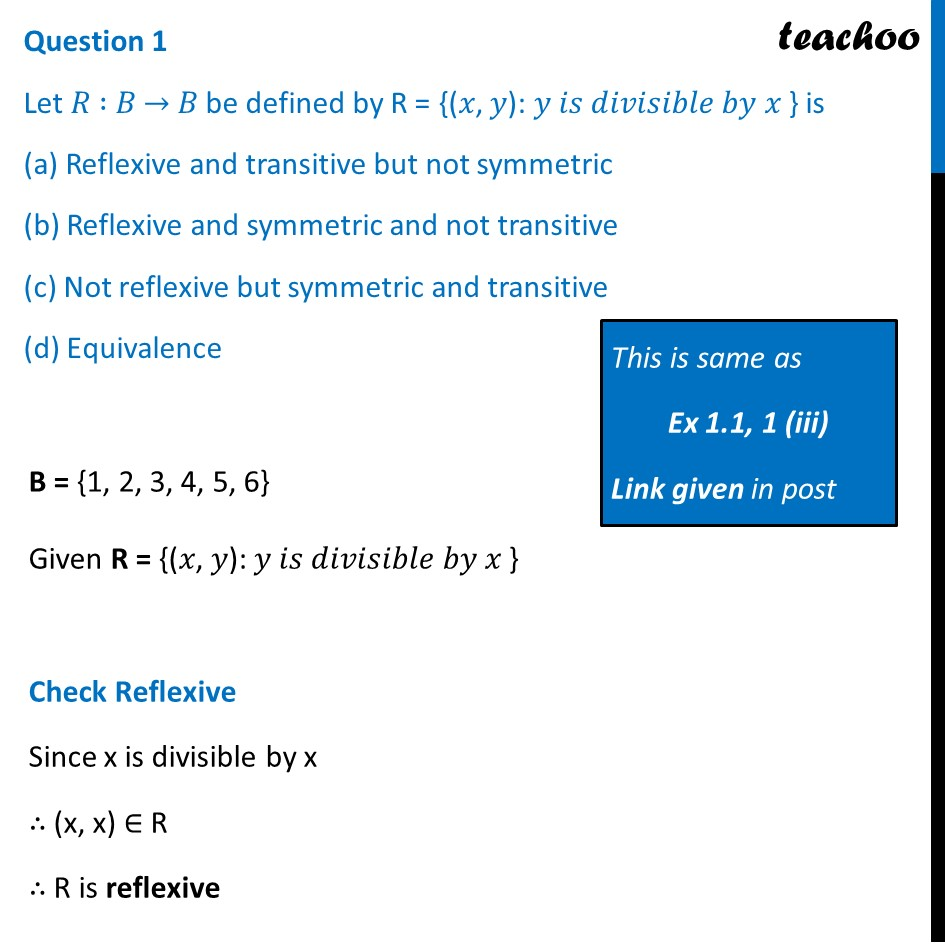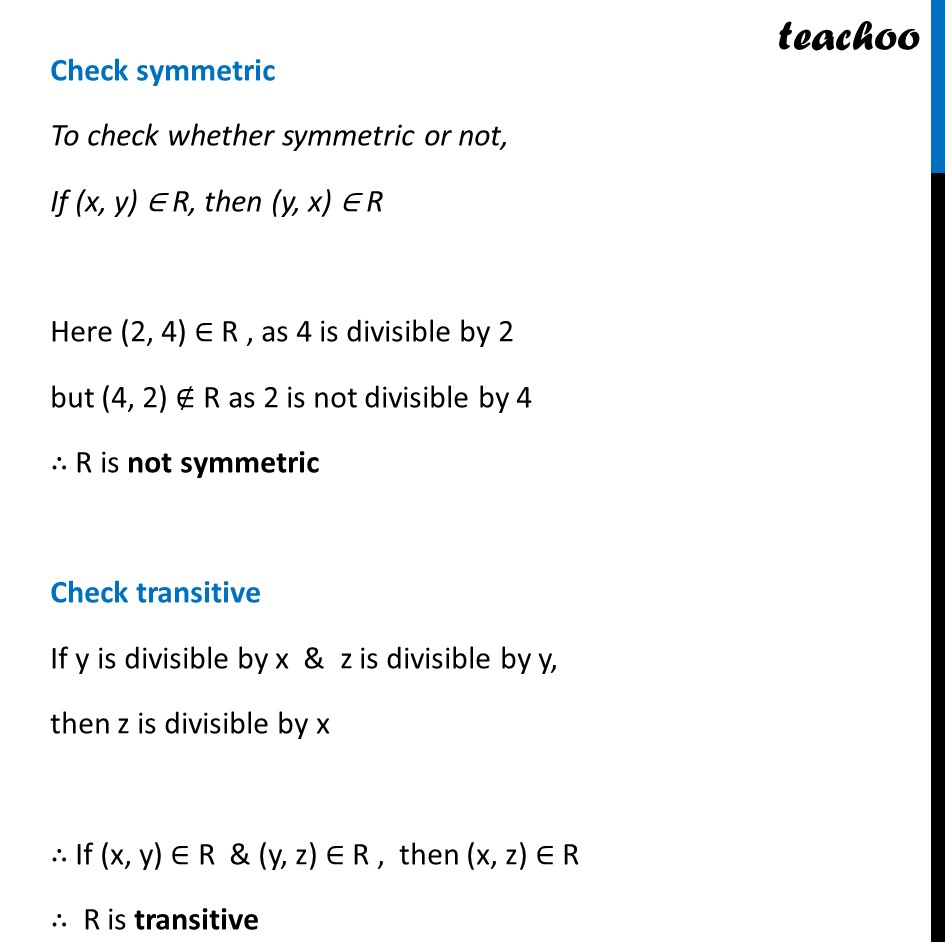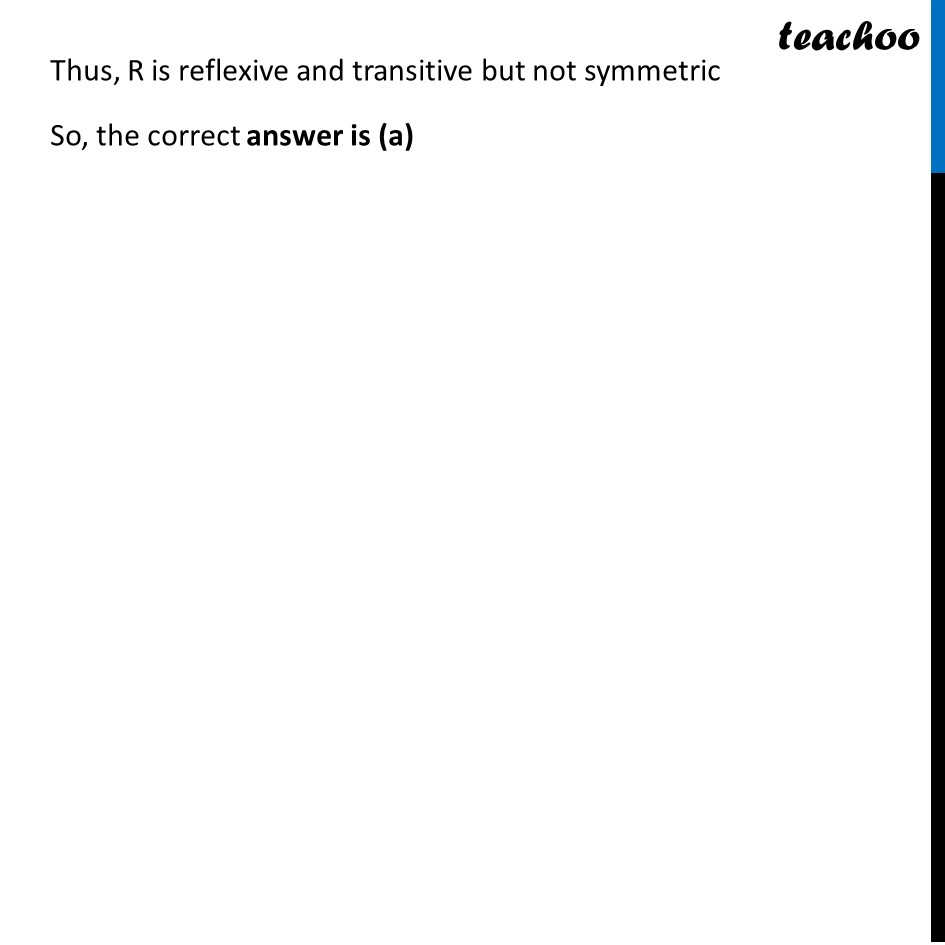## Raji wants to know the number of functions from A to B. How many number of functions are possible? (a) 6 2   (b) 2 6   (c) 6!   (d) 2 12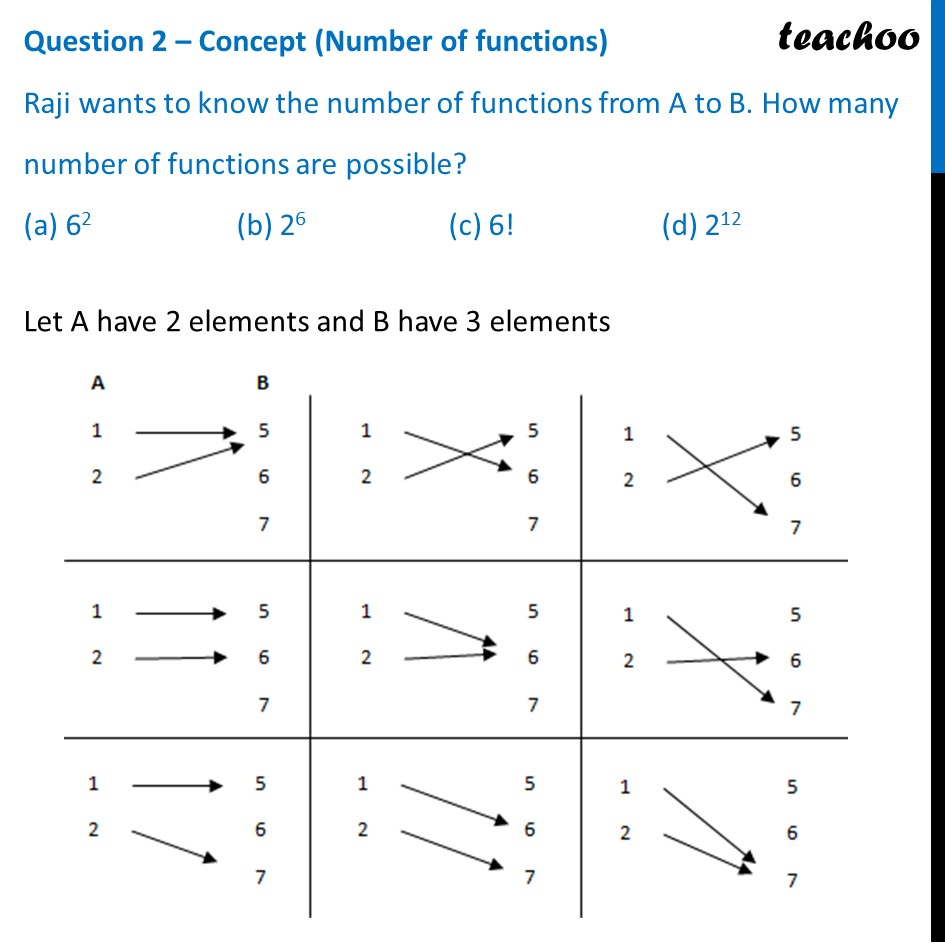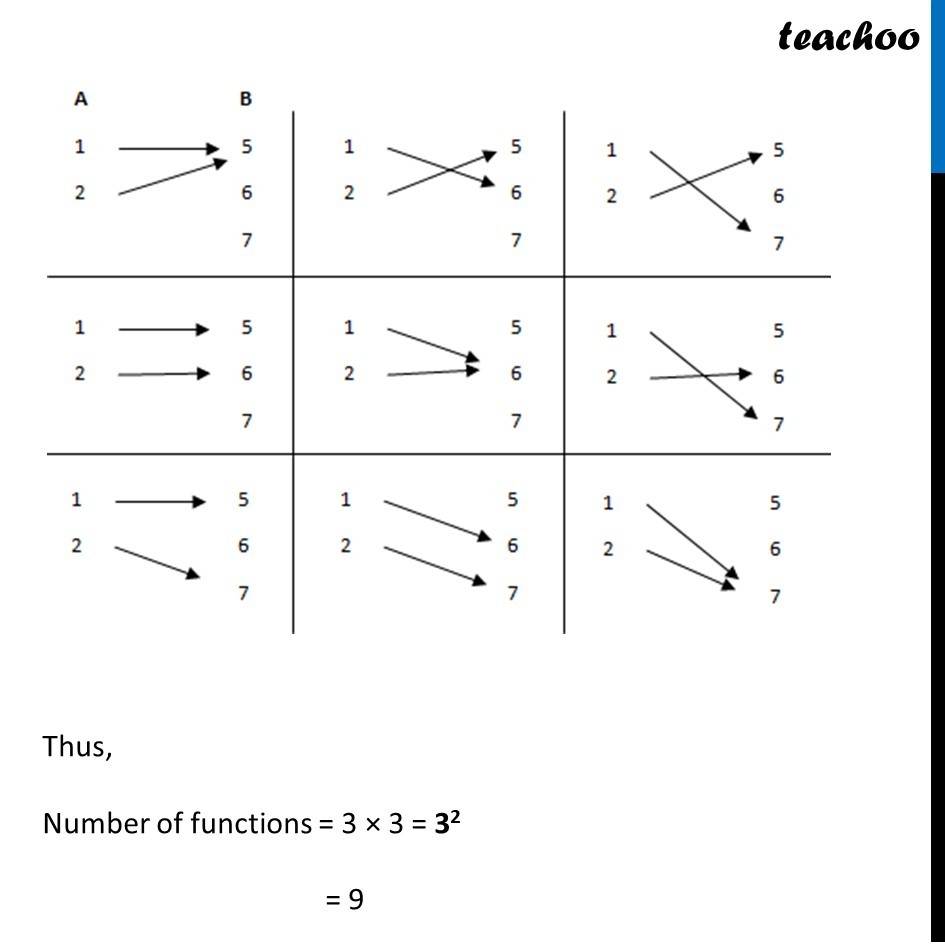## Raji wants to know the number of functions from A to B. How many number of functions are possible? (a) 6 2   (b) 2 6   (c) 6!   (d) 2 12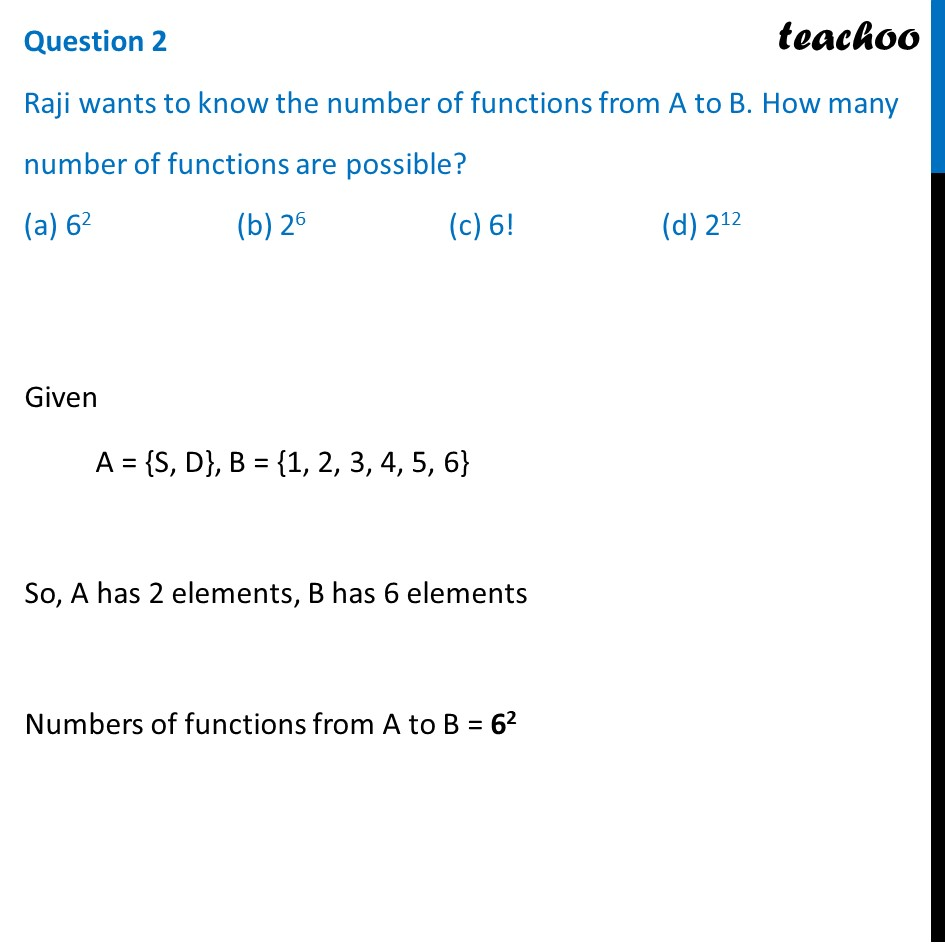## Let R be a relation on B defined by R = {(1, 2), (2, 2), (1, 3), (3, 4), (3, 1), (4, 3), (5, 5)}. Then R is (a) Symmetric (b) Reflexive (c) Transitive (d) None of these three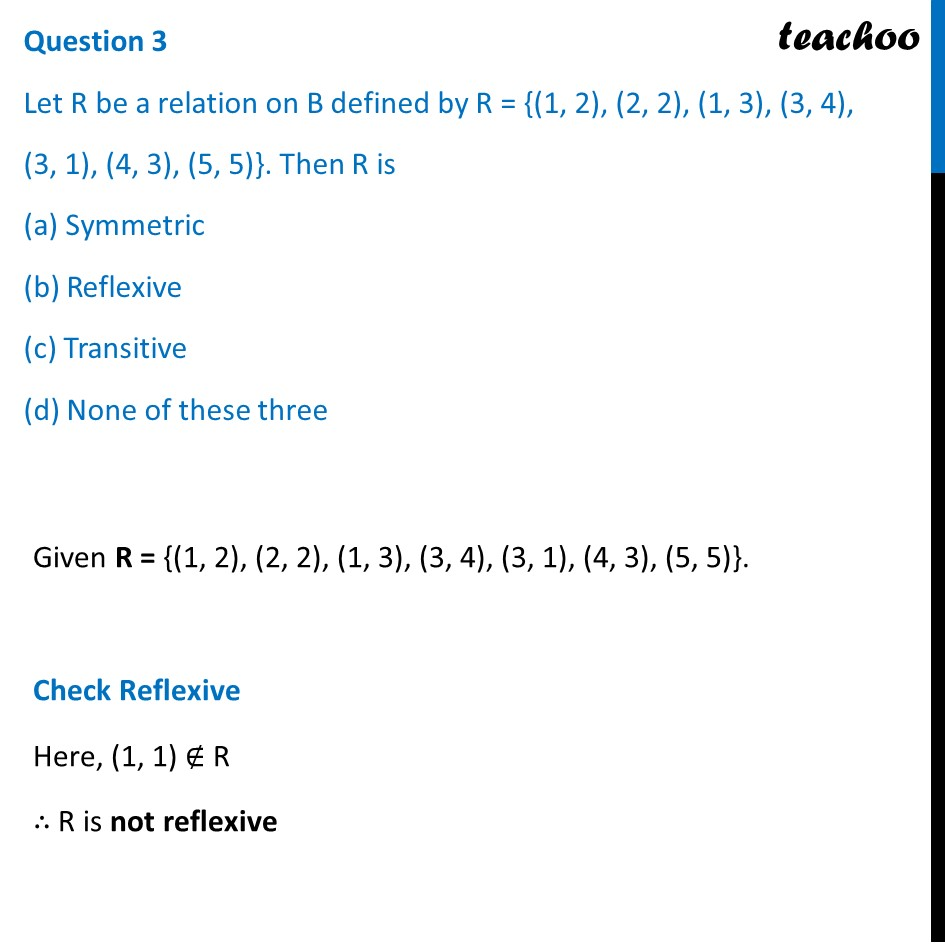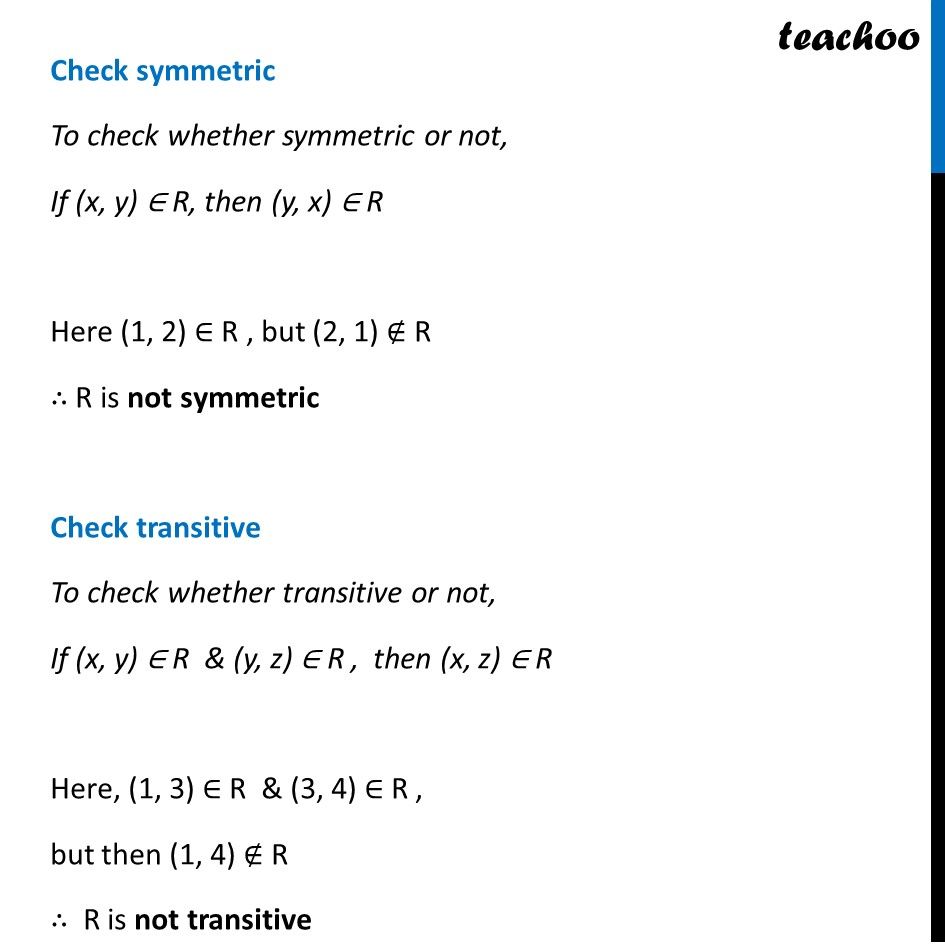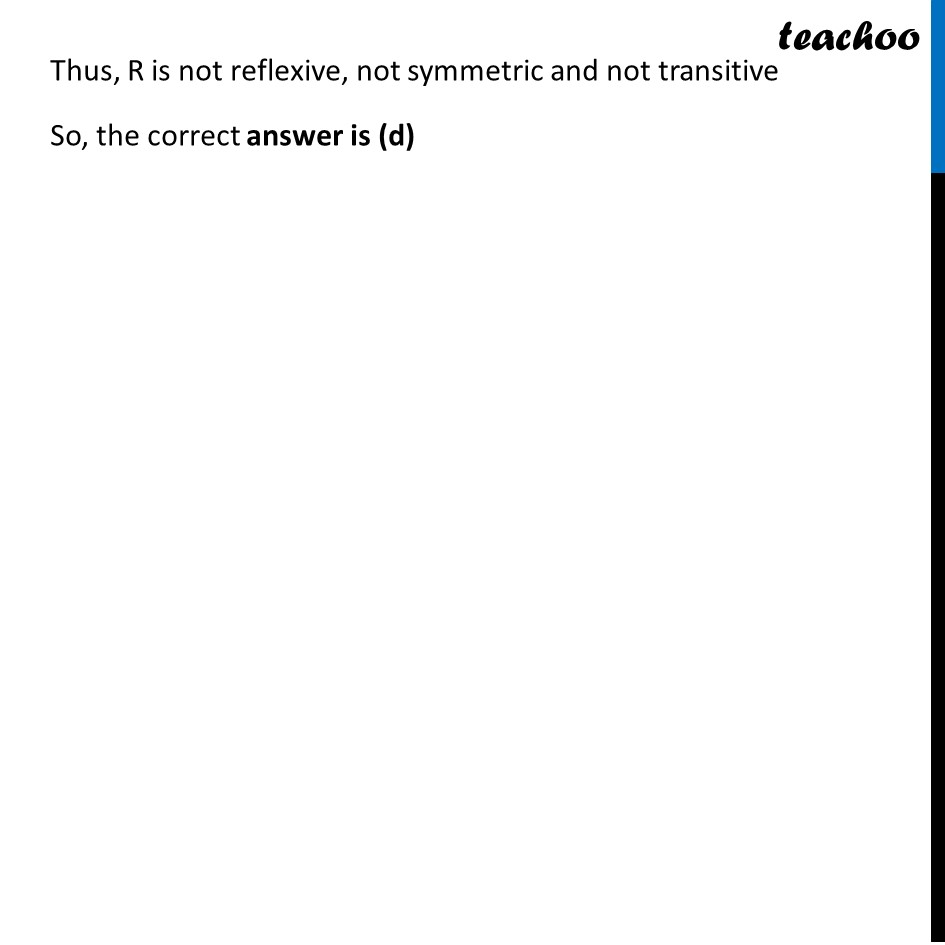## Raji wants to know the number of relations possible from A to B. How many numbers of relations are possible? (a) 6 2   (b) 2 6   (c) 6!   (d) 2 12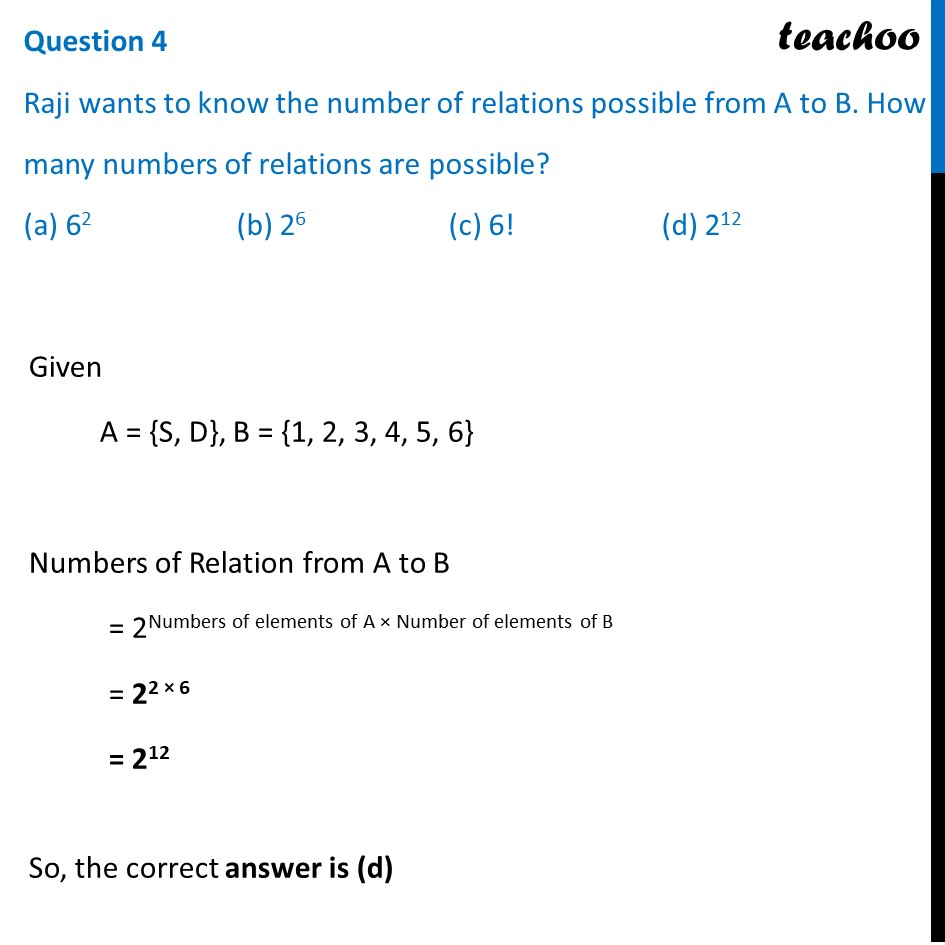## Let 𝑅: 𝐵 → 𝐵 be defined by R = {(1, 1), (1, 2), (2, 2), (3, 3), (4, 4), (5, 5), (6, 6)}, then R is (a) Symmetric (b) Reflexive and Transitive (c) Transitive and symmetric (d) Equivalence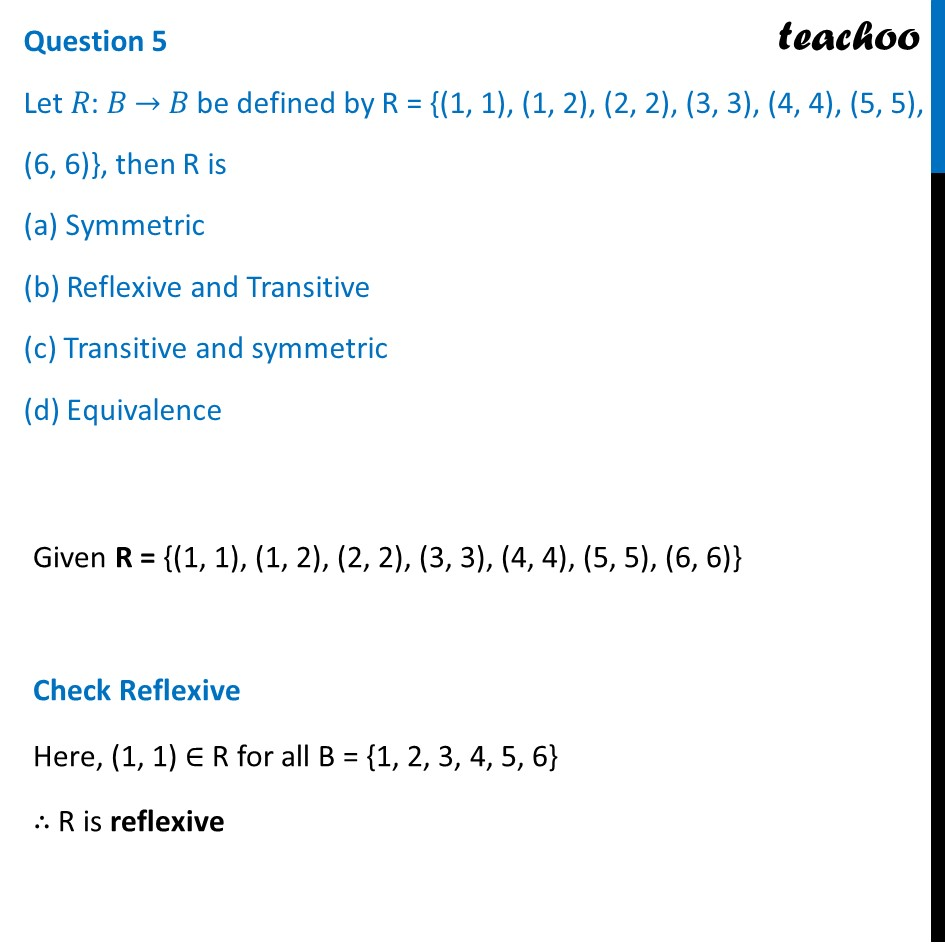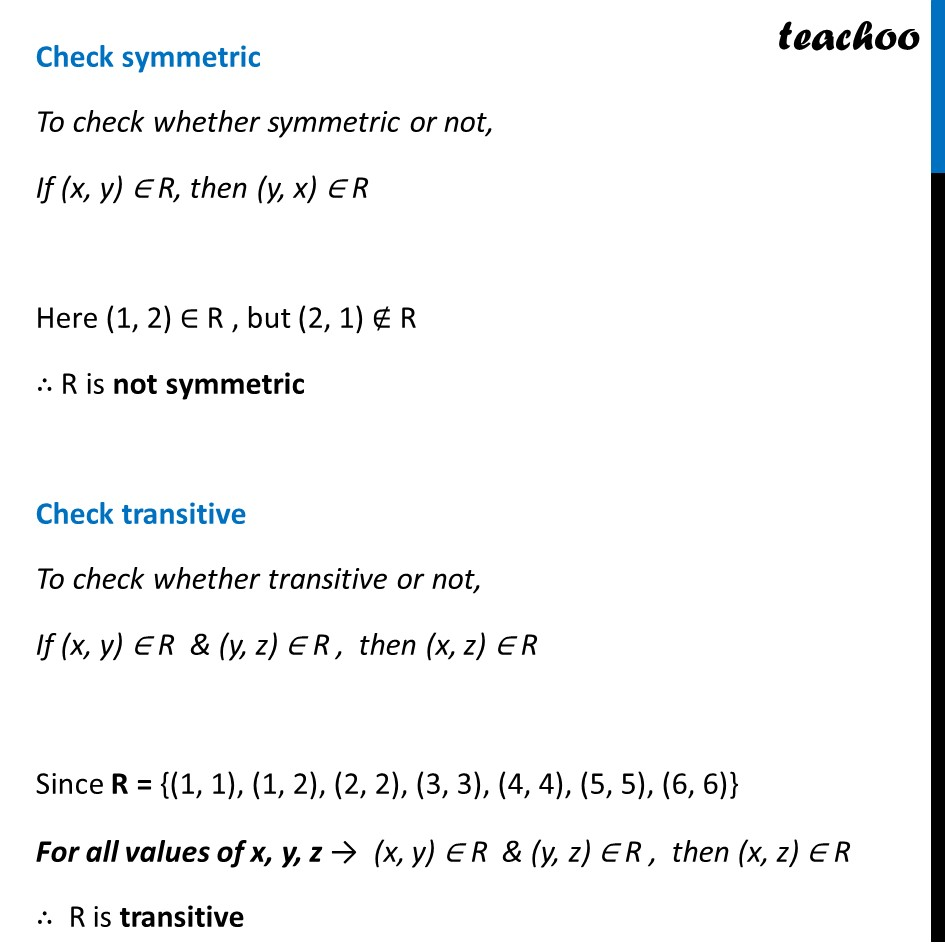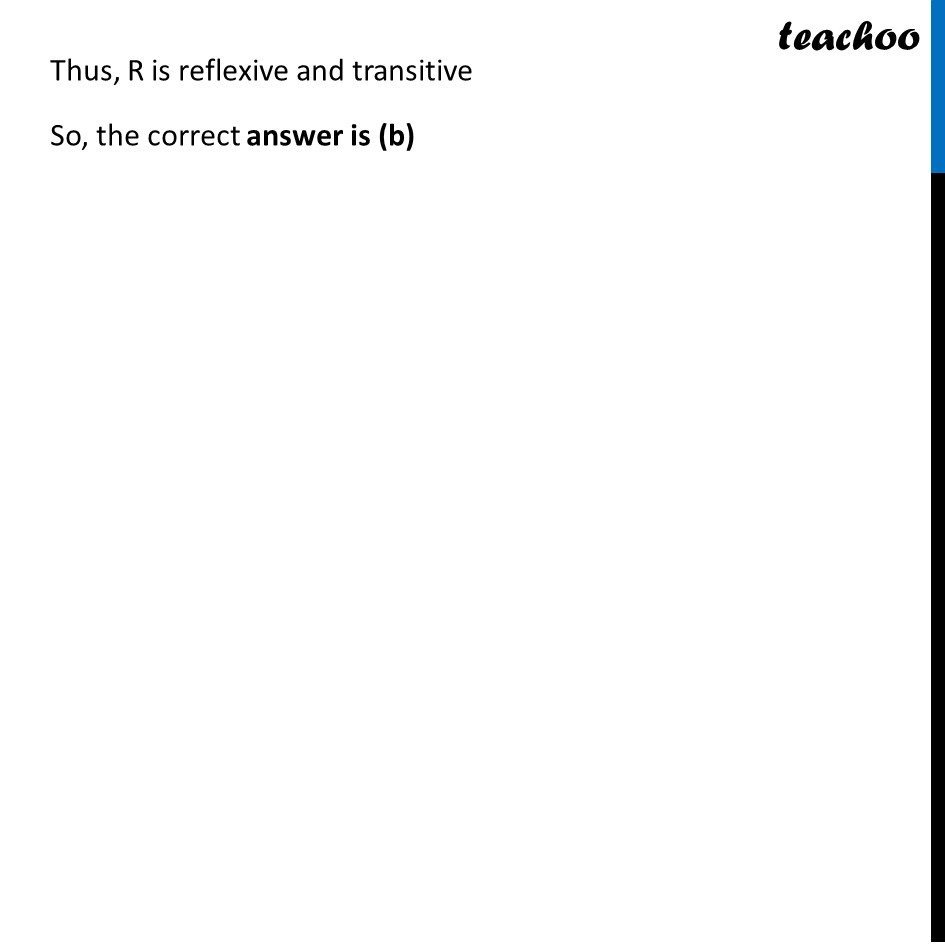1. Chapter 1 Class 12 Relation and Functions (Term 1)
2. Serial order wise
3. Case Based Questions (MCQ)

Transcript

Question Sherlin and Danju are playing Ludo at home during Covid-19. While rolling the dice, Sherlin’s sister Raji observed and noted the possible outcomes of the throw every time belongs to set {1, 2, 3, 4, 5, 6}. Let A be the set of players while B be the set of all possible outcomes. A = {S, D}, B = {1, 2, 3, 4, 5, 6} Question 1 Let 𝑅 ∶ 𝐵 → 𝐵 be defined by R = {(𝑥, 𝑦): 𝑦 𝑖𝑠 𝑑𝑖𝑣𝑖𝑠𝑖𝑏𝑙𝑒 𝑏𝑦 𝑥 } is (a) Reflexive and transitive but not symmetric (b) Reflexive and symmetric and not transitive (c) Not reflexive but symmetric and transitive (d) Equivalence B = {1, 2, 3, 4, 5, 6} Given R = {(𝑥, 𝑦): 𝑦 𝑖𝑠 𝑑𝑖𝑣𝑖𝑠𝑖𝑏𝑙𝑒 𝑏𝑦 𝑥 } Check Reflexive Since x is divisible by x ∴ (x, x) ∈ R ∴ R is reflexive Check symmetric To check whether symmetric or not, If (x, y) ∈ R, then (y, x) ∈ R Here (2, 4) ∈ R , as 4 is divisible by 2 but (4, 2) ∉ R as 2 is not divisible by 4 ∴ R is not symmetric Check transitive If y is divisible by x & z is divisible by y, then z is divisible by x ∴ If (x, y) ∈ R & (y, z) ∈ R , then (x, z) ∈ R ∴ R is transitive Thus, R is reflexive and transitive but not symmetric So, the correct answer is (a) Question 2 – Concept (Number of functions) Raji wants to know the number of functions from A to B. How many number of functions are possible? (a) 62 (b) 26 (c) 6! (d) 212 Let A have 2 elements and B have 3 elements Thus, Number of functions = 3 × 3 = 32 = 9 Question 2 Raji wants to know the number of functions from A to B. How many number of functions are possible? (a) 62 (b) 26 (c) 6! (d) 212 Given A = {S, D}, B = {1, 2, 3, 4, 5, 6} So, A has 2 elements, B has 6 elements Numbers of functions from A to B = 62 Question 3 Let R be a relation on B defined by R = {(1, 2), (2, 2), (1, 3), (3, 4), (3, 1), (4, 3), (5, 5)}. Then R is (a) Symmetric (b) Reflexive (c) Transitive (d) None of these three Given R = {(1, 2), (2, 2), (1, 3), (3, 4), (3, 1), (4, 3), (5, 5)}. Check Reflexive Here, (1, 1) ∉ R ∴ R is not reflexive Check symmetric To check whether symmetric or not, If (x, y) ∈ R, then (y, x) ∈ R Here (1, 2) ∈ R , but (2, 1) ∉ R ∴ R is not symmetric Check transitive To check whether transitive or not, If (x, y) ∈ R & (y, z) ∈ R , then (x, z) ∈ R Here, (1, 3) ∈ R & (3, 4) ∈ R , but then (1, 4) ∉ R ∴ R is not transitive Thus, R is not reflexive, not symmetric and not transitive So, the correct answer is (d) Question 4 Raji wants to know the number of relations possible from A to B. How many numbers of relations are possible? (a) 62 (b) 26 (c) 6! (d) 212 Given A = {S, D}, B = {1, 2, 3, 4, 5, 6} Numbers of Relation from A to B = 2Numbers of elements of A × Number of elements of B = 22 × 6 = 212 So, the correct answer is (d) Question 5 Let 𝑅: 𝐵 → 𝐵 be defined by R = {(1, 1), (1, 2), (2, 2), (3, 3), (4, 4), (5, 5), (6, 6)}, then R is (a) Symmetric (b) Reflexive and Transitive (c) Transitive and symmetric (d) Equivalence Given R = {(1, 1), (1, 2), (2, 2), (3, 3), (4, 4), (5, 5), (6, 6)} Check Reflexive Here, (1, 1) ∈ R for all B = {1, 2, 3, 4, 5, 6} ∴ R is reflexive Check symmetric To check whether symmetric or not, If (x, y) ∈ R, then (y, x) ∈ R Here (1, 2) ∈ R , but (2, 1) ∉ R ∴ R is not symmetric Check transitive To check whether transitive or not, If (x, y) ∈ R & (y, z) ∈ R , then (x, z) ∈ R Since R = {(1, 1), (1, 2), (2, 2), (3, 3), (4, 4), (5, 5), (6, 6)} For all values of x, y, z → (x, y) ∈ R & (y, z) ∈ R , then (x, z) ∈ R ∴ R is transitive Thus, R is reflexive and transitive So, the correct answer is (b)

Case Based Questions (MCQ)